Connect with us

# Process Costing System for Manufacturing CompanyPublished

on

### Process Cost System for Manufacturing Company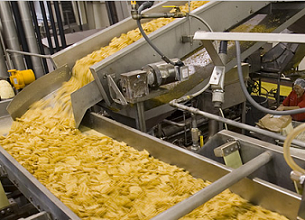In the “Process Costing System“, basically, manufacturers continually process their product, moving it from one function to the next until it is completed. In these companies, the manufacturing costs incurred are allocated to the proper functions or departments within the factory process rather than to specific products. Examples of products that companies produce continuously are cereal, bread, candy, steel, automotive parts, chips, and computers. Companies that refine oil or bottle drinks and companies that provide services such as mail sorting and catalog order are also examples of continuous, homogeneous processing.

1. Flow of The Process Costing System

To illustrate, assume the Lie Dharma Fringles Company manufactures potato chips. The company has three work areas they call preparation, baking, and packaging. The preparation area includes cutting potatoes and adding flavorings. Conveyor belts are used to move the product from one function to the next. In this company, raw materials are added in two of the functions: the preparation function and the packaging function. Labor and overhead are incurred in each function.

Here is the process flow and costs associated with Lie Dharma Fringle’s process cost system: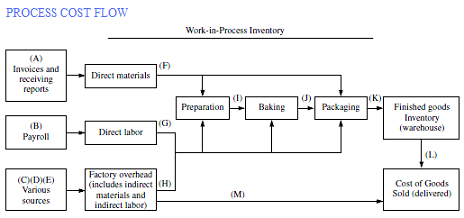2. Cost Summary

The cost report for Lie Dharma Fringles summarizes how manufacturing costs (direct materials, direct labor, and manufacturing overhead) are assigned to the three departments. The report for July is as follows: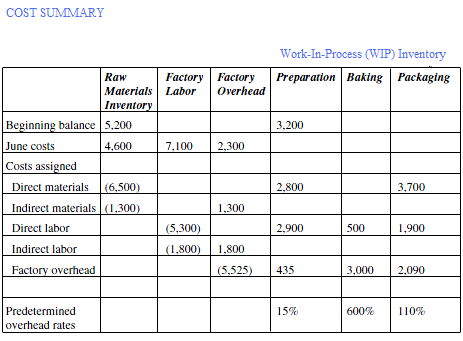As it is sown on the above flow, the process is started from 3 cost components (1) Raw Materials Requisition, (2) Factory Labor, and (3) and Factory Overhead. The raw materials are assigned based on material requisition forms, the labor based on time tickets, and the overhead based on predetermined overhead rates based on direct labor dollars.

The journal entries to record these transactions are made prior to the period end entries that transfer the amounts from one work-in-process inventory account to another, from work-in-process inventory to finished goods inventory, and from finished goods inventory to cost of goods sold.

Note: The letters of the journal entries used to illustrate the accounting for process cost systems (see Key to the process flow above) correspond to the letters.

1. Raw Materials Requisitioned

Lie Dharma Fringles started the month of July with \$5,200 in raw materials inventory. Lie Dharma Fringles uses the perpetual inventory method, so raw materials purchased are added to the raw material inventory account when they are received. Raw materials requisitioned that become part of the final product or are used by a specific function are considered direct materials used. The costs of direct materials are added to the proper department’s work-in-process inventory account. Raw materials requisitioned that are used for general production purposes are added to factory overhead. The journal entries related to raw material activity for July are:

(A). Purchased raw materials on credit

[Debit]. Raw Materials Inventory = 4,600

[Credit]. Accounts Payable = 4,600

(C). Indirect materials for July:

[Credit]. Raw Materials Inventory = 1,300

(F). Transfer direct materials to work-in-process inventory:

[Debit]. Work-in-Process Inventory-Preparation = 2,800

[Debit]. Work-in-Process Inventory-Packaging = 3,700

[Credit]. Raw Materials Inventory = 6,500

At the end of the month, \$2,000 of materials remained in raw materials inventory: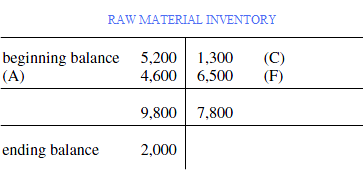2. Factory Labor Cost

As the factory labor payroll is prepared and recorded, the payroll costs are split between those employees who work in specific functions (departments) and those involved in the general functions of the factory. The specific function costs are called direct labor and are assigned to work-in-process inventory. The general factory labor costs are indirect labor costs that are added to factory overhead.

Comparing it to the accounting for payroll under the job order cost system, the employee does not have to be physically involved in making a product to be assigned to a specific function. If a specific maintenance worker or supervisor is assigned to the preparation function, their wages are allocated to that function even though these workers are not directly involved in preparing the chips to be baked. The accounting for the labor costs for July includes the following journal entries, shown below:

(B). Record payroll for July:

[Debit]. Factory Labor = 7,100

[Credit]. Wages Payable = 7,100

(D). Indirect labor for July:

[Credit]. Factory Labor = 1,800

(G). Direct labor for July:

[Debit]. Work-in-Process Inventory-Preparation 2,900

[Debit]. Work-in-Process Inventory-Baking 500

[Debit]. Work-in-Process Inventory-Packaging = 1,900

[Credit]. Factory Labor = 5,300

The balance in the factory labor account should be zero at the end of each period (see below T-account)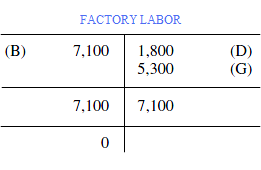In a process company, factory overhead represents those costs not directly assigned to one function. For example, the depreciation expense of a machine used solely by the preparation function would be assigned to work-in-process inventory for the preparation department while depreciation expense for the plant (the factory building) would be assigned to factory overhead as all functions occupy the plant. The journal entries that follow illustrate the accounting for general overhead costs:

[Credit]. Prepaid Insurance = 1,000

[Credit]. Accounts Payable-Electric Utilities = 1,200

[Credit]. Cash = 100

(H). Overhead allocated based predetermined rates:

[Debit]. Work-in-Process = 435

[Debit]. Inventory-Preparation Work-in-Process = 3,000

[Debit]. Inventory-Baking Work-in-Process = 2,090

[Credit]. Inventory-Packaging Factory Overhead = 5,525

At the end of the period, the factory overhead account has a credit balance of (\$125). This is called “Over-applied Overhead” and an entry would be made at the end of the period to move it to cost of goods sold, or alternatively, to allocate the difference to work-in-process inventories, finished goods inventory, and cost of goods sold. After recording this entry, the balance in the factory overhead account is zero.

[Credit]. Cost of Goods Sold = 125

4. Work-In-Process (WIP) Accounting

At the end of the period, entries are needed to record the cost of the products moved from one function (department) to another. In this example, costs are moved from work-in-process inventory-preparation to work-in-process inventory-baking and from work-in-process inventory-baking to work-in-process inventory-packaging. This is how the entries would look:

(I). Transfer July costs to Baking:

[Debit]. Work-in-Process Inventory-Baking = XXX

[Credit]. Work-in-Process Inventory-Preparation = XXX

(J). Transfer July costs to Packaging:

[Debit]. Work-in-Process Inventory-Packaging = XXX

[Credit]. Work-in-Process Inventory-Baking = XXX

When the packaging function (department) completes its work, the product is ready to be sold. The costs of the completed products are then transferred from work-in-process inventory-packaging to finished goods inventory. This transfer also requires a journal entry:

(K). Transfer to finished goods inventory:

[Debit]. Finished Goods Inventory = XXX

[Credit]. Work-in-Process Inventory-Packaging = XXX

The amounts for these journal entries are calculated by multiplying the cost per unit times the number of units that moved from one function to the next. The number of units is determined separately for each function using the actual number of units completed and transferred out of the function adjusted for partially completed units that were not transferred. This calculated number of units used is called equivalent units. If there are no in-process units at the beginning or end of the period, the “per unit cost” is calculated by dividing the total costs assigned to a function (department) by the total number of units that were started and completed during the period. The total costs include materials, labor, and overhead.

Per unit cost of function =

[Total costs for function in period] / [Total units started and completed]

If the function has work-in-process inventory at the beginning of the period, the number of equivalent units must be calculated. Equivalent units represent the number of units that could have been 100% completed during the period. For example: if two employees each work 20 hours a week, this is the equivalent of one full-time employee (one equivalent unit). On a production line, if one product is 40% complete and a second one is 60% complete, this is the equivalent of 100% complete for one unit (one equivalent unit). This number is needed to spread the costs of the function over all the units worked on during the period. For example, if a company started 1,000 units of product during the period and at the end of the period these were 40% completed, the equivalent units would be 400 (1,000 units × 40% complete).

This calculation assumes that the materials, labor, and overhead are all added evenly throughout the time the units are in process in the function. In many companies, the materials are all added at the beginning of the process while the labor and overhead costs are incurred throughout the process. Labor and overhead are also called conversion costs because they “convert” the materials into a product. If materials, labor, and overhead are added at different times in the production process, two separate calculations of equivalent units are necessary, one for the materials and one for conversion costs.

Using the previous example of 1,000 units started during the period that were 40% completed, assume that in a particular function, all of the materials are added at the beginning of the process and the labor and overhead are added evenly throughout the process. The equivalent units for materials would be the number of units times the percent complete. In this example, all the materials are added at the beginning of the process so 100% of materials for this function are included in all the units at the end of the period. The equivalent units for materials are 1,000 (=1,000 units × 100% complete for materials). The total materials costs are divided by 1,000 to calculate the materials cost per unit.

Unlike materials, more labor and overhead will be needed before these units are transferred to another function or to finished goods. The equivalent units for conversion costs (labor and overhead) are 400 (=1,000 units started × 40% complete for labor and overhead). The total conversion costs are divided by 400 to calculate the conversion costs per unit. To calculate total cost per unit, the materials cost per unit is added to the conversion cost per unit.

When a company has units that are started and completed during a period and has an ending inventory of units in process, most often the weighted average method is used to calculate equivalent units. If needed, based on the company’s production processes, separate calculations of equivalent units for materials and conversion costs are made. Assume a company has two functions in its production process called Department 1 and Department 2. For the month of January, Department 1 completed and transferred out 2,000 units to Department 2 and had 800 units in process at month end that were 80% completed as to materials, labor, and overhead. Using the weighted average method, equivalent units for Department 1 for January are 2,640 [=(2,000 × 100%) + (800 × 80%)]. The beginning units and those started and completed are not separately identified in the calculation of equivalent units. When calculating the per unit cost using the weighted average method, the beginning work-in-process costs for the function are added to those costs incurred during the period and then divided by the equivalent units.

Equivalent units may also be calculated using the first-in, first-out (FIFO) method. Under the FIFO method of calculating equivalent units, the beginning units would be identified separately from those started and completed. Continuing with the previous example, if 700 units were in process and 40% completed at the beginning of January, during January Department 1 would have added the additional 60% of the costs necessary to complete the units. Using the FIFO method, the equivalent units for January would be 3,060, calculated as follows: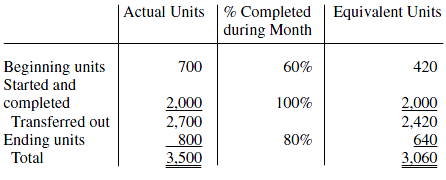5. Process Costing Summary

Once the physical units have been identified and the equivalent units calculated, the per unit cost is calculated and the cost summary is prepared for each function. Assume the following facts and costs for Department 1 for September. Overhead costs are based on direct labor hours.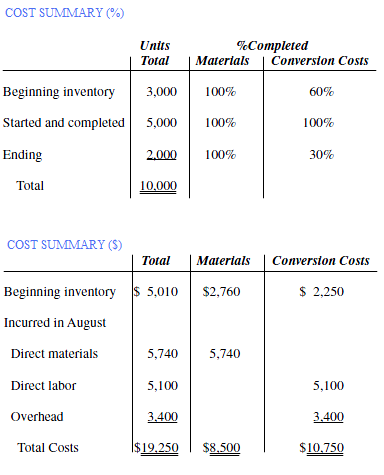The next 2 figures show the process cost summary for Department 1 using the previous information for September under the weighted average and FIFO methods, respectively. The summary includes sections for the flow of the units, equivalent units, unit costs for the period, costs to be allocated (costs to be accounted for), and allocation of costs to the units transferred out and those units in process at the end of the period (costs accounted for).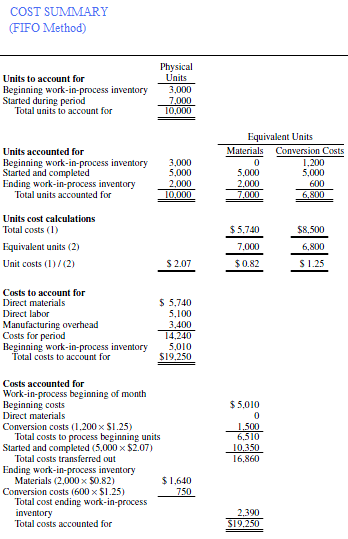In the weighted average example, the calculation of number of units accounted for does not differentiate between units in beginning inventory and those units started and completed during the period because the costs are averaged for all these units. The per unit costs are based on the equivalent units completed and the total costs incurred on those units. Under the FIFO method, costs are tracked based on specific units. Per unit costs are current period unit costs and calculated based on equivalent units completed and costs incurred for the current period. For the units in the beginning inventory, once the current period costs are calculated, they are added to the costs incurred in prior periods to determine the total costs for these units.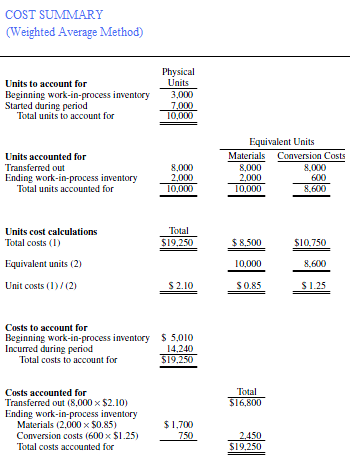Traditionally, in “Process Costing System“, overhead is allocated to a function based on direct labor hours, machine hours, or direct labor dollars. However, in some companies, new technologies have changed the manufacturing environment such that the number of hours worked or dollars earned by employees are no longer good indicators of how much overhead will be needed to complete a job or process products through a particular function. In such companies, activity-based costing is used to allocate overhead costs to jobs or functions. Activity-based costing (well abbreviated as ABC system) provides a way to allocate costs more accurately when overhead costs are not incurred at the same rate as direct labor dollars.

Pages: 1 2

Are you looking for easy accounting tutorial? Established since 2007, Accounting-Financial-Tax.com hosts more than 1300 articles (still growing), and has helped millions accounting student, teacher, junior accountants and small business owners, worldwide.